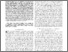# Frequency domain non-linear compact modelling and simulation of IC spiral inductors on silicon

Brinson, Mike (2018) Frequency domain non-linear compact modelling and simulation of IC spiral inductors on silicon. International Journal of Microelectronics and Computer Science, 9 (1). pp. 19-26. ISSN 2080-8755Preview
Text
IJMCS_MEB_paper_2018.pdf - Accepted Version

## Abstract / Description

SPICE AC circuit simulation is fundamentally a small signal network analysis of a linear or non-linear circuit operating at speciﬁed DC bias conditions, where the electrical network component values are assumed not to be functions of AC input signal frequency. In the case of RF circuit simulation this assumption can give rise to signiﬁcant modelling errors.With the recent improvements in General Public License (GPL) circuit simulators this situation is changing, particularly through the introduction of Frequency Dependent Equation-Deﬁned Device (FEDD) models, non-linear current/voltage static and dynamic Equation-DeﬁnedDevice(EDD)modelsandusercontrolledswept signal frequency simulation employing Harmonic Balance steady state analysis. The main purpose of this paper is to introduce a number of novel modelling and circuit simulation techniques that allow, and enhance, the construction of compact device models with embedded behavioural components whose non-linear properties are functions of AC input signal frequency. To demonstrate these new modelling techniques a compact model for a 10 GHz band width spiral inductor integrated on silicon is introduced, its compact model presented, and ﬁnally its simulation performance compared with published measured device data.

Item Type: Article Qucs, Qucs-S, Ngspice, Xyce, compact device modelling, Frequency Equation-Defined Devices (FEDD), Equation-Defined Devices (EDD), SPICE B type sources, Harmonic Balance simulation 600 Technology600 Technology > 620 Engineering & allied operations School of Computing and Digital Media Bal Virdee 22 Oct 2018 08:51 01 Apr 2022 12:42 https://repository.londonmet.ac.uk/id/eprint/3552View Item